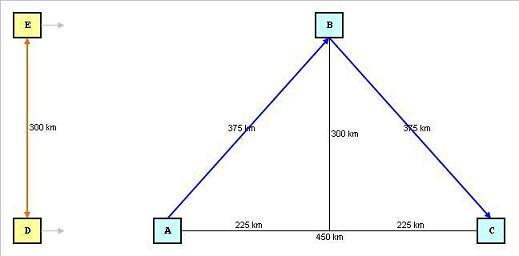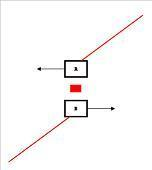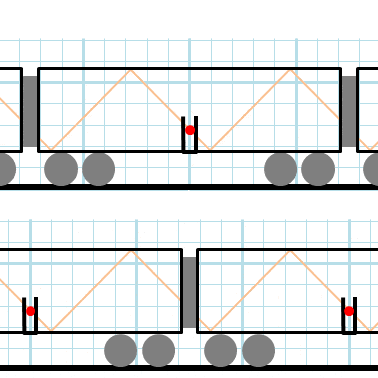# Torch Contraction Problem in SR: A and B Observe Differently

• B
• Stephanus

#### Stephanus

Dear PF Forum,
There's a problem in SR that I cannot solve (or understand)This is, I think, a well known SR problem.
The picture is telling the problem itself.

A and B and C are in the same frame (let's call it ABC frame)
D and E are in the same frame (DE)

wrt DE
D stays and E stays above D, 300 km.
ABC are approaching DE.
When A meets D, D shines a light PERPENDICULAR above it and when the light reaches E, E meets B.
E then bounces the light vertically down. When the light reaches D again, D meets C.

wrt ABC
ABC sees that DE are approaching ABC and the light shines diagonally not vertically.
D meets with C and then later, A ask C what its clock reading when C meets D. C says 2.5 seconds
Because A knows that C is 450 km away, then A will know DE speed.

I think in SR, A doesn't have to ask C to know DE speed.
Somehow the universe has arranged it? I mean like Twin paradox, the planet doesn't have to really see that the rocket has turned around. It's sufficient that the rocket worldline has crossed planet worldline then the planet experience time dilation.
I'm sorry I can't express my point clearly. I hope you understand what I mean in the paragraph above.

Here is my problem
ABC will see the light travels diagonally, ED will see the light travels vertically. If we do the "simple" math, we'll find that the distance and time will be in Lorentz factor ##\gamma = \frac{1}{\sqrt{1-v^2}} = 1.25##
But how can ABC sees the light diagonally? What if ABC insist that they stay and it's DE who are moving?
This is permitted in SR, right. So the solution is, it's not a beam of light. Light expands in sphere. The beam of light that ABC see traveling diagonally, will be seen by DE vertically. The beam of light that ABC see traveling vertically, will be seen by DE diagonally.
It's just that particular beam that ABC sees diagonally and seen by DE vertically that makes C sees D clock is time dilated. Of course D will see ABC clocks time dilated as well. That C clock is 2.5 seconds and D clock is 2 second, it's the relative simulatenity of events as understood by D.
That I think I have already understood above.
But what if D sends a laser beam? E will agree that E receives high intensity light. Proof that the light is vertical wrt DE.
Now, B will receive a high intensity light won't he? This both party would agree.
E and B receive high intensity light.

My question
1. How can DE see the same light as seen by ABC vertical, while that same light travels diagonally according to ABC?
2. Shouldn't the light not affected by the motion?
3. I think it's the same whether DE bring the laser transmitter or the laser transmitter is lying around on the ground, and DE and ABC fly from different direction and when DE and ABC meets, won't they see the laser hits vertically above them?
4. I think in no 3, they both will see the laser shines diagonaly5. Does length contraction in this case play a role in the length of the torch or emitter. Where a stationary observer will see that the torch/emitter somehow tilted and causing the light/signal to travel diagonally?

Thanks you.

Last edited:
You're confusing a very simple matter. Imagine someone is on a train, throwing a ball up and down. To them it goes straight up and down: from point D to E and back down to D . But, to someone watching the train go past, the ball will go up and down in a curve: the ball will be thrown from point A, reach point B and be caught at point C. Tha ball does not need to be thrown "at a diagonal".

Your real problem here is not SR or light or length contraction of time dilation; it's a basic understanding of two reference frames, moving with respect to each other. The motion of an object or a light ray must be different in the two frames.

•Ibix
I'm in a train and it's raining. The streaks of rain on the window point towards the back of the train. Everyone agrees this, but they don't agree why. To me, the train is stationary and the rain is falling on a diagonal. To an observer on a platform the rain is falling vertically but the train moves while the drop is in the process of striking it.

The same thing is happening with the light pulses. Some people describe them as moving diagonally, some as moving vertically. They are different descriptions of the same thing.

I think I can see where you are going wrong. You wrote about your first diagram that the two experiments were in different frames. No. A frame is not a thing that you can be in or not - a thing is in all frames. A frame is just a point of view. You have drawn two pictures of the same experiment taken from different points of view - one moving with respect to the experiment and one not. Just like the rain and the train. And just like the rain and the train, if you look closely at the workings then you will find two different descriptions of every bit of the process, linked by the Lorentz transforms, that explain why each perspective sees what it sees.

A laser is a pair of mirrors with a gain medium between. In the frame where it is stationary the mirrors repeatedly reflect photons bouncing vertically up and down, so it is reasonable to expect the beam to come out vertically. In the frame where it is moving the mirtors reflect photons moving diagonally so we expect the beam to come out diagonally. It's the same photons, just a different description of what they're doing.

From an old post of mine:
Maybe this diagram helps:Here's something (it could be a pulse of light, it could be a ball) bouncing up and down in a train.

An observer in the train (top) infers the thing is moving vertically up and down.

An observer on the ground (bottom) infers the thing is "sliding sideways" in a zig-zag path.

This is valid in both relativistic and Newtonian mechanics.

•m4r35n357 and PeroK
Thank you @PeroK
Thank you @Ibix
Thank you @DrGreg
What wonderful answers. Really I appreciate it.

What confuses me is this.
If a light source (turned off) is moving toward West, East, myself, North and South.
The light source travels from west to east, 0.6 c
The distance between West, East, myself north and south is 360 km

When the light source meets me, it shines.
So the light will reach East not in 0.75 ms (v is not 480 000 km/s) but in 1.2 ms. (v = 300 000 km/s) according to this ##w = \frac{c+v}{1+cv} = \frac{1+v}{1+1v} = 1##
And the light will reach West not in 3 ms (v is not 120 000 km/s) but also in 1.2 ms ##w = \frac{v-c}{1+-cv} = \frac{v-1}{1- v} = -1##

Let me compare it with bullet or ball or pebble.
A plane (180 m/s) fires a missile (300 m/s) the speed of missile 400 m/s
A rocket (180 000 km/s) shines a light (300 000 km/s) the speed of light 300 000 km/s unaffected

Now a plane (180 m/s) fires a missile (300 m/s above) the speed of the missile is ##\sqrt{180^2+300^2} = 349.857 m/s##
Missile vertical speed: 300 m/s
Missile horizontal speed 180 m/s

A rocket (180 000 km/s) shines a light (300 000 km/s above) the speed of light is ##\sqrt{180000^2+v^2} = 300000 km/s; v = \sqrt{300000^2 - 180000^2} = 240000km/s##
Light vertical speed: 240,000 km/s
Light horizontal speed 180,000 km/s

So light horizontal speed is unaffected for light that is shone to east/west
However vertical speed is affected.
At first I though the direction of light is unaffected by the source movement, but it is affected I think.

Thank you.

At first I though the direction of light is unaffected by the source movement, but it is affected I think.
The speed is unaffected. The direction can be affected.

•Stephanus
So light horizontal speed is unaffected for light that is shone to east/west
However vertical speed is affected.
At first I though the direction of light is unaffected by the source movement, but it is affected I think.
I'm really not sure about your maths, because I don't think I've completely grasped any of the scenarios you are tring to describe. However you are correct that, with the exception of light moving in the ±x direction, a Lorentz boost in the x direction will change the components of the velocity. So, observed from a different frame, the light moves at the same speed in a different direction.

•Stephanus
Thank you @PeroK
Thank you @Ibix
Thank you @DrGreg
What wonderful answers. Really I appreciate it.

What confuses me is this.
If a light source (turned off) is moving toward West, East, myself, North and South.
The light source travels from west to east, 0.6 c
The distance between West, East, myself north and south is 360 km

When the light source meets me, it shines.
So the light will reach East not in 0.75 ms (v is not 480 000 km/s) but in 1.2 ms. (v = 300 000 km/s) according to this ##w = \frac{c+v}{1+cv} = \frac{1+v}{1+1v} = 1##
And the light will reach West not in 3 ms (v is not 120 000 km/s) but also in 1.2 ms ##w = \frac{v-c}{1+-cv} = \frac{v-1}{1- v} = -1##

Let me compare it with bullet or ball or pebble.
A plane (180 m/s) fires a missile (300 m/s) the speed of missile 400 m/s
A rocket (180 000 km/s) shines a light (300 000 km/s) the speed of light 300 000 km/s unaffected

Now a plane (180 m/s) fires a missile (300 m/s above) the speed of the missile is ##\sqrt{180^2+300^2} = 349.857 m/s##
Missile vertical speed: 300 m/s
Missile horizontal speed 180 m/s
By using your equation, you get an answer of 349.857113690718... m/s. The correct answer is 349.857113690672... m/s, which ends up giving a vertical speed for the missile of 299.999999999946 m/s As measured by someone watching the plane fly by at 180 m/s. If we bump up the speed of plane and missile by a factor of 10,000 (3,000,000 & 1,800,000 m/s), then the total speed of the missile becomes ~3499946 m/s and its vertical speed becomes ~2,999,946 m/s as measured by the same person.
The point is that you have to use Relativistic velocity addition for orthogonal velocities just like you do for parallel ones.
A rocket (180 000 km/s) shines a light (300 000 km/s above) the speed of light is ##\sqrt{180000^2+v^2} = 300000 km/s; v = \sqrt{300000^2 - 180000^2} = 240000km/s##
Light vertical speed: 240,000 km/s
Light horizontal speed 180,000 km/s

So light horizontal speed is unaffected for light that is shone to east/west
However vertical speed is affected.
At first I though the direction of light is unaffected by the source movement, but it is affected I think.

Thank you.

•Stephanus
At first I though the direction of light is unaffected by the source movement, but it is affected I think.
You are getting dangerously close to discussing aberration of light.
Another source is here, starting about half way down. Look for "Meanwhile, Einstein's 1905 paper on the electrodynamics of moving bodies . . ."
The maths might (or not) be a challenge, but those are the answers that I think you are looking for.

•Stephanus
Thank you @Ibix
Thank you @Janus
Thank you @A.T.
Thank you @m4r35n357

I'm really not sure about your maths, because I don't think I've completely grasped any of the scenarios you are tring to describe.
Actually, it's simple.
Someone holding a torch vertically moves toward you.
I was wrong about the "light" concept which for me it's not a light concept, rather heavy I thinkIn parallel direction.

A plane 100 m/s fires a missile 300 m/s horizontally
The speed of the missile, wrt:
Plane: 300 m/s
Rest observer: 400 m/s (well actually, it's 399.9999... something). Changed!

A rocket 100 000 km/s shoot a laser 300 000 km/s horizontally
The speed of the laser, wrt
Rocket: 300 000 km/s
Rest obs: 300 0000 km/s. Not changed!

And this is where I go wrong.

A plane 100 m/s fires a missile 300 m/s vertically
The speed of the missile,
wrt Plane:
V Horizontal: 0 m/s
V Vertical: 300 m/s
V total: 300 m/s

wrt Rest observer:
V Horizontal: 100 m/s, Changed!
V Vertical: 300 m/s
V Total: ##\sqrt{100^2+300^2} = 316.2278##

A rocket 100 000 km/s shoot a laser 300 000 km/s vertically
The speed of the laser,
wrt Rocket:
V Horizontal: 0 m/s
V Vertical: 300 000m/s
V total: 300 000m/s

wrt Rest observer:
V Horizontal: 0 m/s, Not Changed, I was wrong here
V Vertical: 300 000 m/s
V Total: 300 000 km/s
Okay.. I know the calculation. But I was wrong

It should be like this:
V Horizontal: 100 000 m/s
V Vertical: ##\sqrt{300000^2-100000^2} = 282,843 km/s##
V Total: 300 000 km/s

The speed is unaffected. The direction can be affected.
Thank you very much. Just as in ball thrown vertically in the train, right? I thought the speed of light is not changed, so the direction is not changed either. I was wrong.

By using your equation, you get an answer of 349.857113690718... m/s. The correct answer is 349.857113690672... m/s, which ends up giving a vertical speed for the missile of 299.999999999946 m/s As measured by someone watching the plane fly by at ...
Thank you very much Janus. What about horizontal speed? But I think the answer is in Relativistic for orthogonal. I'll study it by myself. Thanks
The point is that you have to use Relativistic velocity addition for orthogonal velocities just like you do for parallel ones.
Thanks, I'll study it later.

You are getting dangerously close to discussing aberration of light.
Another source is here, starting about half way down. Look for "Meanwhile, Einstein's 1905 paper on the electrodynamics of moving bodies . . ."
The maths might (or not) be a challenge, but those are the answers that I think you are looking for.
Aberration of light? Yeah I've read that before. I will read velocity addition for orthogonal and aberration of light by myself.

Thank you very much, guys.
I know the answer of my question.
The speed is unaffected. The direction can be affected.
And also I have many more new questions. Orthogonal velocity addition, light aberration, etc.

You are getting dangerously close to discussing aberration of light.
Another source is here, starting about half way down. Look for "Meanwhile, Einstein's 1905 paper on the electrodynamics of moving bodies . . ."
The maths might (or not) be a challenge, but those are the answers that I think you are looking for.
Oh, it's you @m4r35n357 . I just saw you relativistic animation video in other thread. A good video.

Oh, it's you @m4r35n357 . I just saw you relativistic animation video in other thread. A good video.
Cheers. I hope you got the point that once you consider more than one spatial dimension (which is what I picked up on), angles of light travel become very important, and change from one frame of reference to another.
This is IMO where SR becomes really cool, as you can do all that visualization stuff with just 2 space dimensions and one of time. This keeps the algebra manageable.
On the other hand if that is not where you are really going with this then I apologize for butting in!

Cheers. I hope you got the point that once you consider more than one spatial dimension (which is what I picked up on), angles of light travel become very important, and change from one frame of reference to another.
This is IMO where SR becomes really cool, as you can do all that visualization stuff with just 2 space dimensions and one of time. This keeps the algebra manageable.
On the other hand if that is not where you are really going with this then I apologize for butting in!
You tell meI only know SR in 1 spatial dimension, non acceleration frame. That's why I haven't understood Bell Paradox, yet.
Thank you very much for you help all this time.
The speed of light is invariant in all frame.
I thought the angle, too. Mea culpa